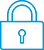cancel
Showing results for
Did you mean:It has been a while since anyone has replied. Simply ask a new question if you would like to start the discussion again.New member
2 1 0 0
Message 1 of 4
998
Flag Post

Solved!

# Integer parameterHP Recommended
Hp prime graphing calculator
Hello there!
I have a big problem, I don' t know how to enter a integer parameter on hp prime calculator!
I enter N (as natural) put it doesn't work
Tags (1)
1 ACCEPTED SOLUTION

Accepted SolutionsLevel 6
305 297 32 76
Message 4 of 4
Flag PostHP Recommended

Okay, now I understand what you meant.

In this case we need the assume command:

assume(k, integer)

See the User Guide information about the assume command, which gives this example.

A method to get to know what the second argument , so in this case "integer", should be is to enter

type(7)

where 7 in an arbitrary integer. The result is DOM_INT, which means we could also have used:

assume(k, DOM_INT)

Now we get:Note that the screen shows TYPE(7), but I entered type(7), so lowercase.

This is a wrong display.

type and TYPE are not the same commands.

type is meant for use in CAS and TYPE for use in Home View.

We now have created a variable k with certain properties.

Do not forget to delete it when you do not need these properties anymore.

You can do this in  the Memory Manager (key B)

Choose CAS Vars.

Tags (1)
3 REPLIES 3Level 6
305 297 32 76
Message 2 of 4
Flag PostHP Recommended

It is unclear to me what you are trying to do.

Maybe you could give a simple example?

When you want to write an equation which contains parameters this can most easily be done in CAS view, so press the CAS key.

We can not use A.....Z because these are special variables which are always replaced by their content, which in this case is always a real number.

But we can use their minor case forms, so a, b.....t, u, z or we can define any variable we like, for example MYvar.

So we could write:

a*x+b

And if we like we can store integers into a and b.

We could also store real numbers or complex numbers into them though.New member
2 1 0 0
Message 3 of 4
Flag PostHP Recommended

I'll try to give you a simple example.

If I Do a Definite integral of cos(kx) dx, the calculator show the result (1/Ķ)sin(kx).

I need the app o button that evaluate the expression for K natural, not for a specific number, but for the all natural number.

The result should be zero.

On others calculators I used @n1 but it doesn't works.

Sorry for my grammar 😞Level 6
305 297 32 76
Message 4 of 4
Flag PostHP Recommended

Okay, now I understand what you meant.

In this case we need the assume command:

assume(k, integer)

See the User Guide information about the assume command, which gives this example.

A method to get to know what the second argument , so in this case "integer", should be is to enter

type(7)

where 7 in an arbitrary integer. The result is DOM_INT, which means we could also have used:

assume(k, DOM_INT)

Now we get:Note that the screen shows TYPE(7), but I entered type(7), so lowercase.

This is a wrong display.

type and TYPE are not the same commands.

type is meant for use in CAS and TYPE for use in Home View.

We now have created a variable k with certain properties.

Do not forget to delete it when you do not need these properties anymore.

You can do this in  the Memory Manager (key B)

Choose CAS Vars.

Tags (1)
† The opinions expressed above are the personal opinions of the authors, not of HP. By using this site, you accept the Terms of Use and Rules of Participation